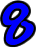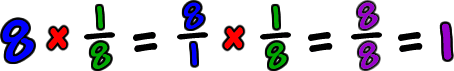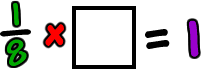Let's look at a number...  8...What number can we multiply to 8 to get 1 (the multiplicative identity) as the answer?Wow!  That's a really weird problem.  Can you think of any integers that would work?  Nope.  So...  It must be a fraction!  Remember that we want 1 for the answer... and 1 in fraction language with 8's isSo, the multiplicative inverse of 8 is 1/8!

Let's go the other way...

What number can we multiply to 1/8 to get 1 as the answer?Remember this!So, the multiplicative inverse of 1/8 is 8!